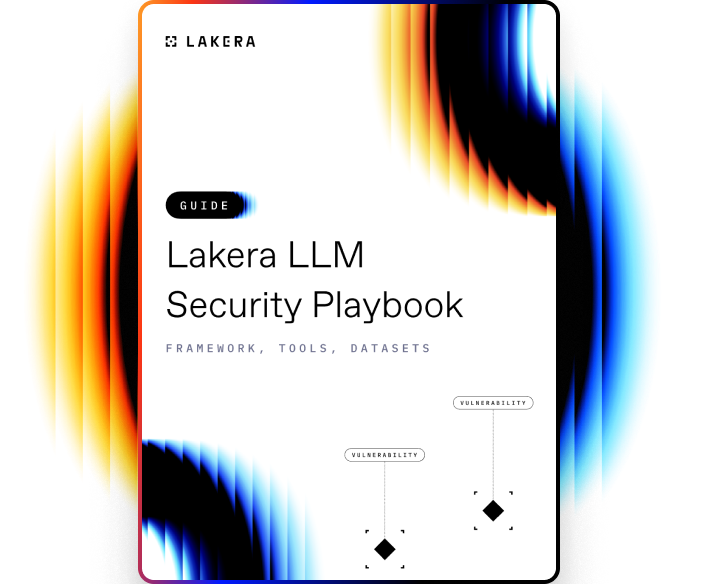VOCABULARY

# Model Training

Model training is a key step in the machine learning process where a mathematical/statistical model learns from a given dataset. This involves feeding the model an input (a dataset) and allowing it to make predictions, and then adjusting the model's parameters based on the accuracy of those predictions.

The ultimate goal is to optimize the model's performance for making future predictions.

## How Model Training works

Model training works as follows:

1. Defining a model: Start by selecting a type of model you want to use (e.g. linear regression, decision tree, neural network, etc.) This decision is primarily based on the type of data you have and the problem you're trying to solve.
2. Initialize parameters: The model's parameters (e.g., weights in a neural network) need to be initialized. These can be adjusted during the learning process.
3. Feed the model with training data: The training data (input) is fed to the model. This data should have both the independent variables (features) and dependent variable (target).
4. Make a prediction: The model uses the input data to make predictions. These predictions are compared to actual outcomes to assess accuracy.
5. Evaluate the prediction: A loss function is used to measure the difference between the model's predictions and the actual values. The aim is to minimize this loss.
6. Adjust the parameters: Based on the prediction error (loss), the parameters of the model are updated using an optimization algorithm (like gradient descent). This optimization process is iteratively done to reduce the difference between the predicted and actual values.
7. Repeat the process: Steps 3 to 6 are repeated until the loss stops reducing significantly, indicating that the model has learned patterns in the data.

Once the model has been trained and the loss is minimal, it can be used to make predictions on unseen or new data. This process is called model testing or validation.

Remember, while training a model, it's important to avoid overfitting (where the model learns too much from the training data and performs poorly on unseen data) and underfitting (where the model doesn't learn enough from the training data and therefore performs poorly even during training).Learn how to protect against the most common LLM vulnerabilities

Download this guide to delve into the most common LLM security risks and ways to mitigate them.

Related termsActivate
untouchable mode.